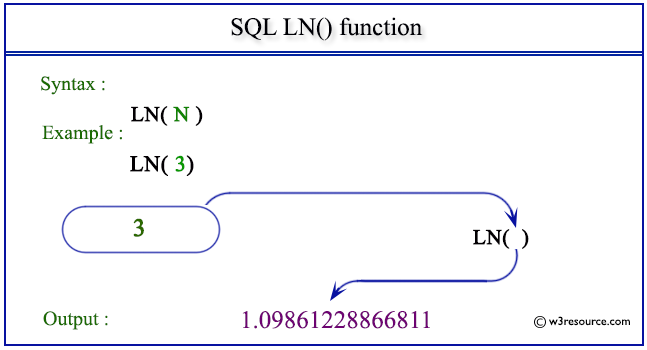# SQL LN() function

## LN() function

SQL LN() function returns the natural logarithm of n, where n is greater than 0 and its base is a number equal to approximately 2.71828183.

Syntax:

` LN(expression) `

DB2, PostgreSQL, and Oracle

All of above platforms support the SQL syntax of LN().

MySQL and SQL Server

If you are using above two platforms, use LOG() instead.

Parameters:

Name Description
expression An expression which is a float or can be converted to a float.

## SQL LN() function: Pictorial presentationExample:

To get the natural logarithm of 65 from the DUAL table, the following SQL statement can be used:

``````SELECT LN(65) "natural_log of 65"
FROM dual;
``````

Output:

```natural_log of 65
-----------------
4.17438727
```

Note: Outputs of the said SQL statement shown here is taken by using Oracle Database 10g Express Edition.

Here is a slide presentation which covers the SQL arithmetic functions.

Practice SQL Exercises

Previous: EXP
Next: MOD

﻿

## SQL: Tips of the Day

Count the number of occurrences of a string in a VARCHAR field?

```SELECT
title,
description,
ROUND (
(
LENGTH(description)
- LENGTH( REPLACE ( description, "value", "") )
) / LENGTH("value")
) AS count
FROM <table>
```

Ref: https://bit.ly/3PvNdT4